# ML | Introduction to Strided Convolutions

Let us begin this article with a basic question – “Why padding and strided convolutions are required?”

Assume we have an image with dimensions of n x n. If it is convoluted with an f x f filter, then the dimensions of the image obtained are.

Example:
Consider a 6 x 6 image as shown in figure below. It is to be convoluted with a 3 x 3 filter. The convolution is done using element wise multiplication.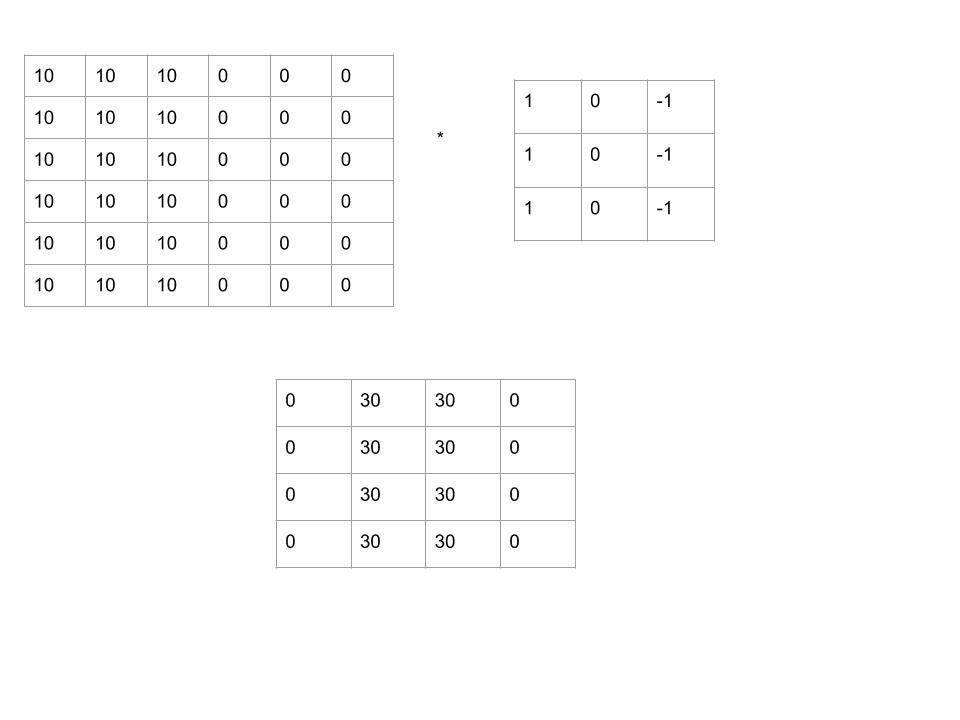Figure 1: Image obtained after convolution of 6×6 image with a 3×3 filter and s=0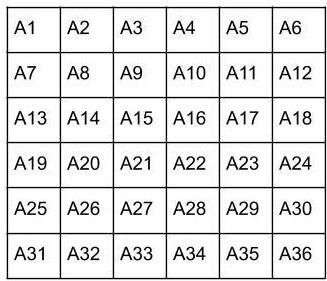Figure 2: 6 x 6 filter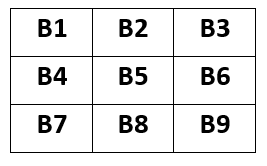Figure 3: 3 x 3 filter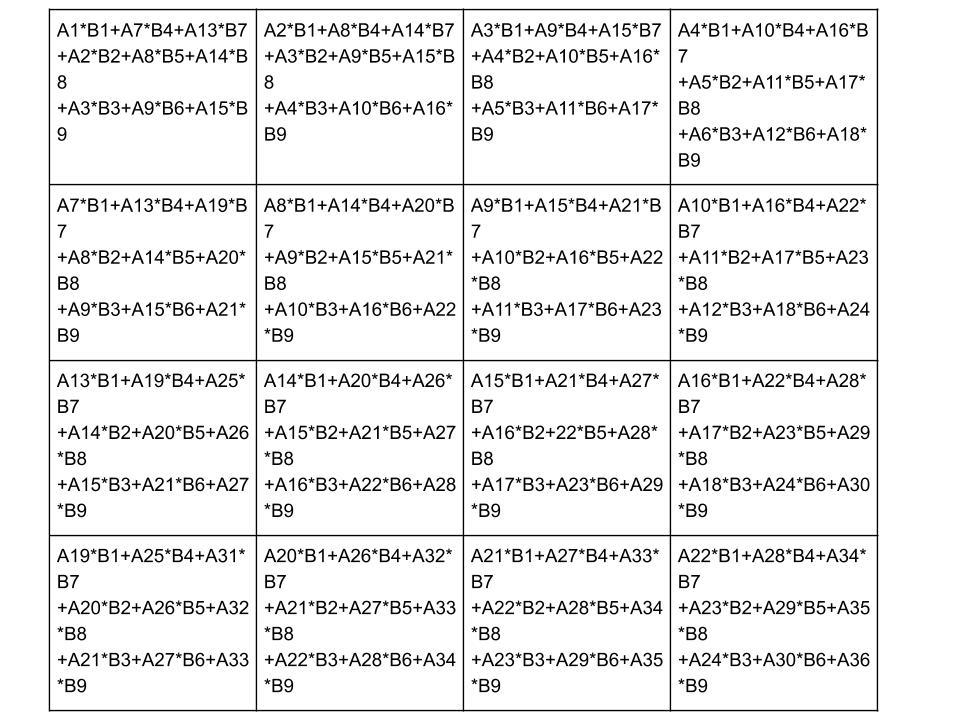Figure 4: Element wise multiplication
But there are two downsides of this convolution:

1. By applying the convolutional filter every time, the original image sinks. i.e. the output image has smaller dimensions than the original input image which may lead to information loss.
2. Pixels at the corner of the image used in only one of the outputs than pixels in the middle which lead to huge information loss.

In order to avoid it, padding is required. Also, sometimes it happens that we have a very large input image is to be convoluted with an f x f filter which may be computationally very expensive. In this situation, strides are used . That is why padding and strides are one of the most basic building blocks of Convolutional Neural Networks

Dimensions of output image :

Lets have an n x n image to be convoluted with an f x f filter. Assume a padding border of p pixels and a stride s, then the dimensions of the output image obtained areThe stride amount should be selected such that comparatively lesser computations are required and the information loss should be minimum.
Figure 5: Image obtained after convolution of 6×6 image with a 3×3 filter and a stride of 2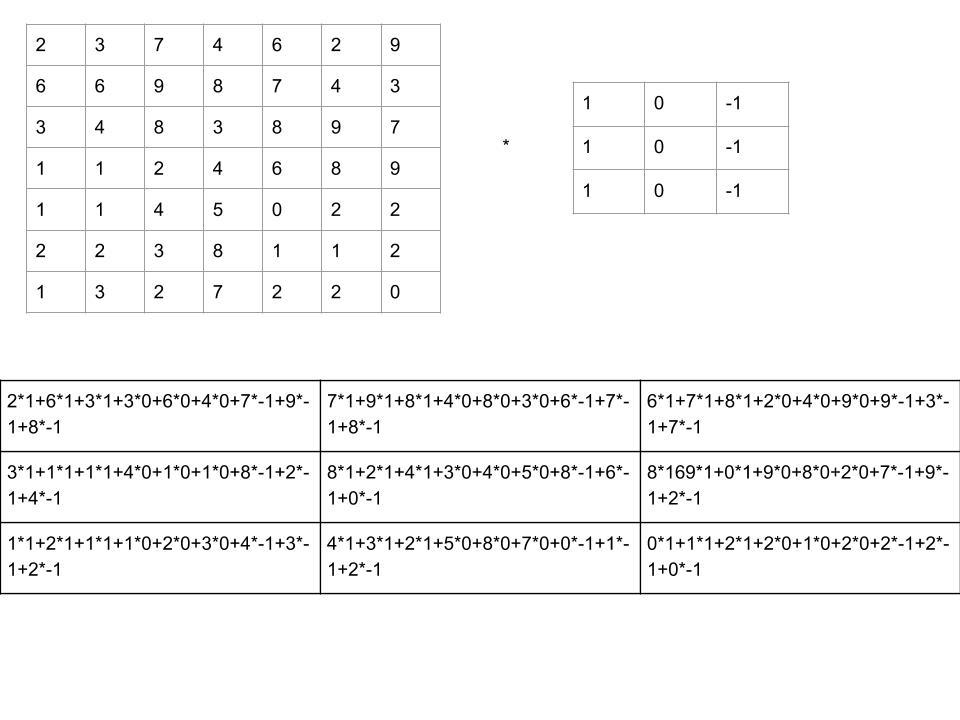Attention reader! Don’t stop learning now. Get hold of all the important DSA concepts with the DSA Self Paced Course at a student-friendly price and become industry ready.

My Personal Notes arrow_drop_upCheck out this Author's contributed articles.

If you like GeeksforGeeks and would like to contribute, you can also write an article using contribute.geeksforgeeks.org or mail your article to contribute@geeksforgeeks.org. See your article appearing on the GeeksforGeeks main page and help other Geeks.

Please Improve this article if you find anything incorrect by clicking on the "Improve Article" button below.